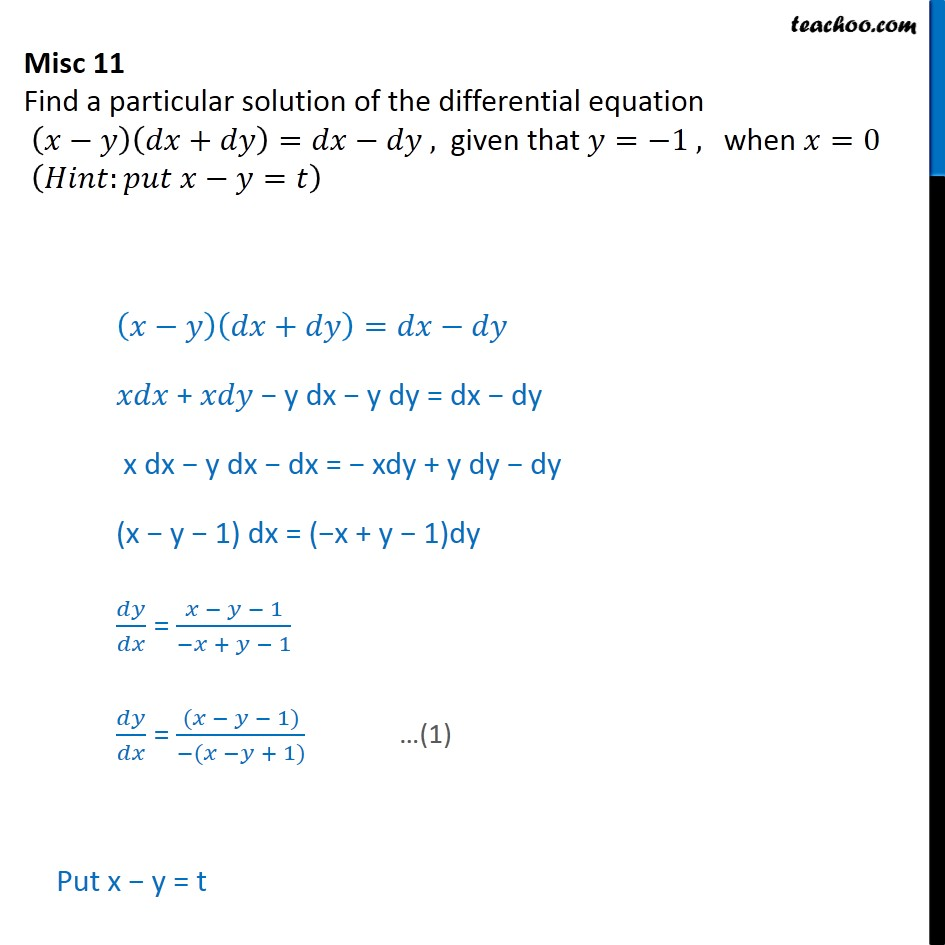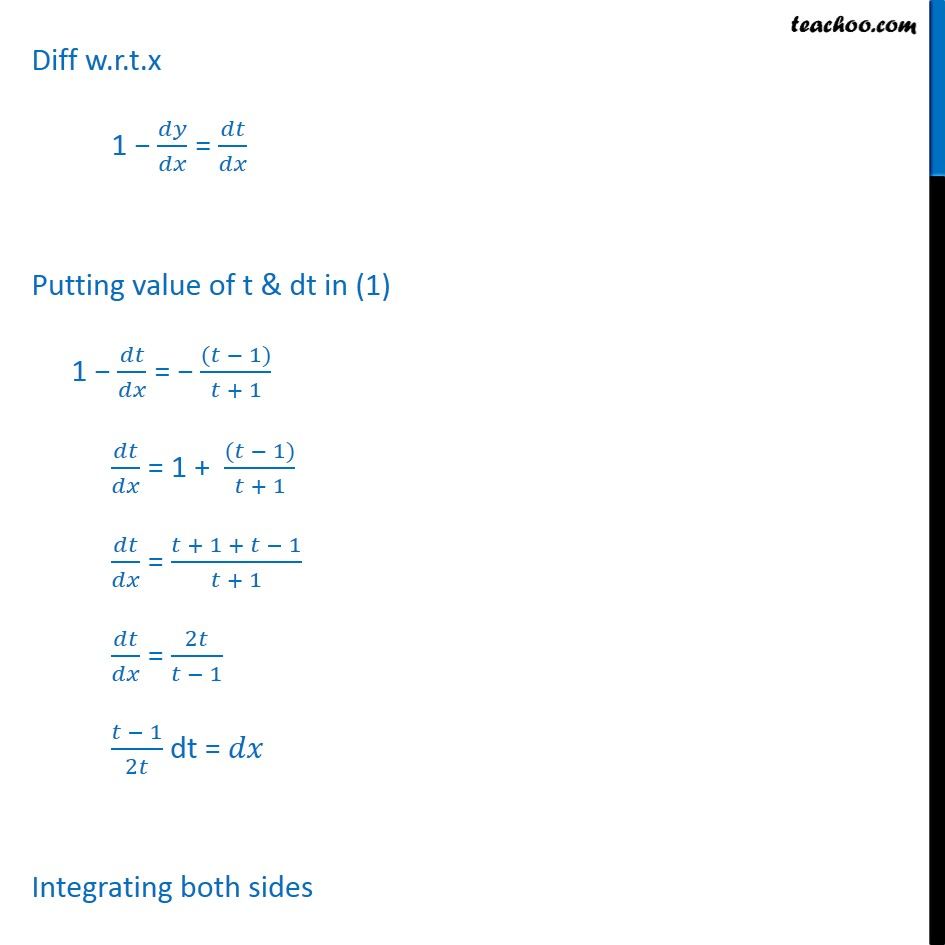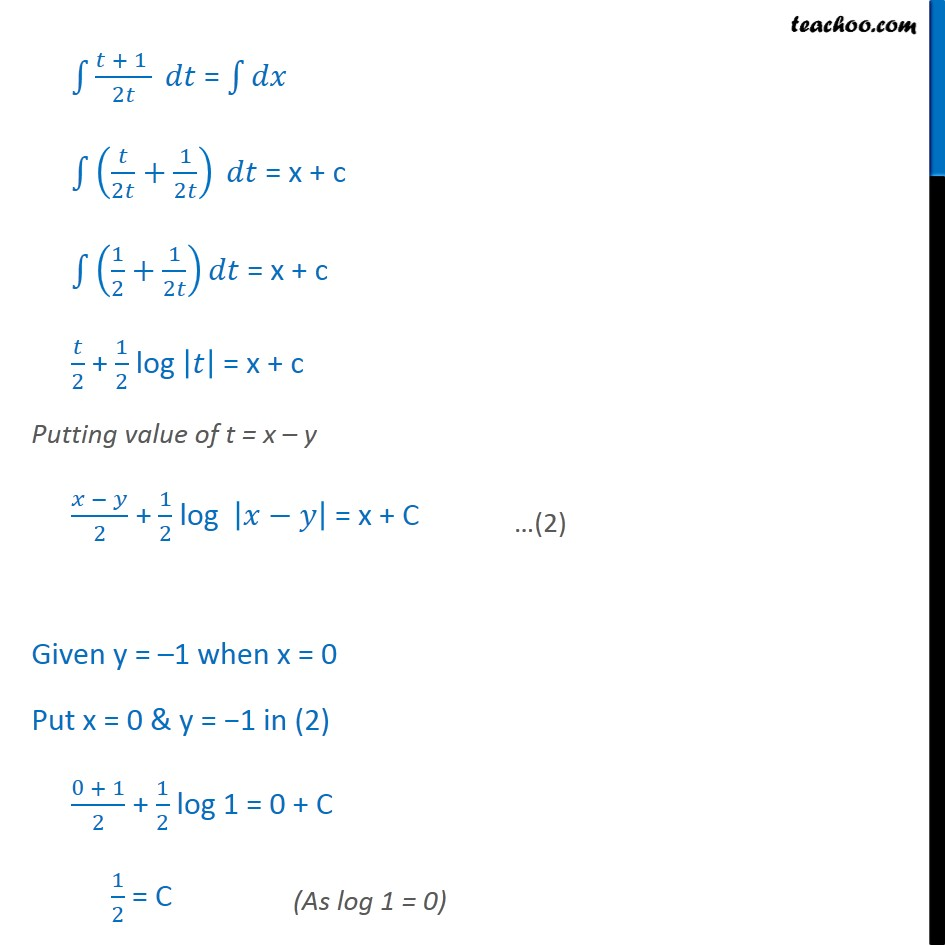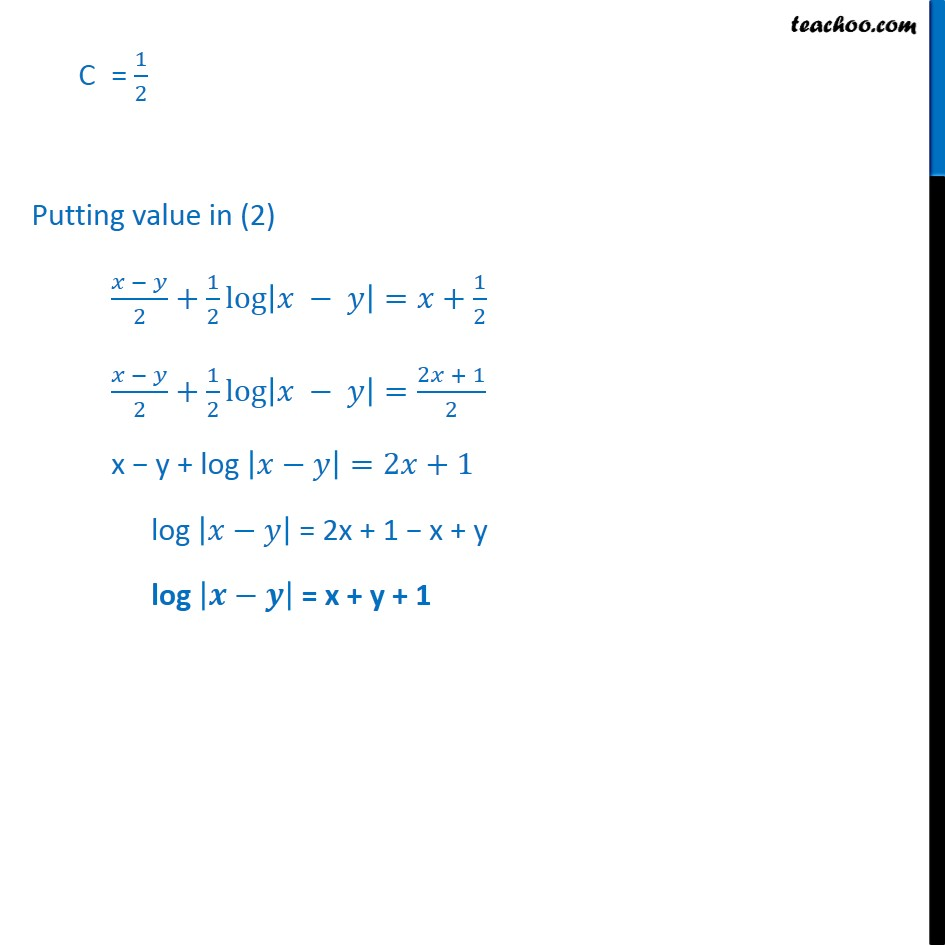Miscellaneous

Chapter 9 Class 12 Differential Equations
Serial order wiseLearn in your speed, with individual attention - Teachoo Maths 1-on-1 Class

### Transcript

Misc 9 Find a particular solution of the differential equation ( )( + )= , given that = 1 , when =0 ( : = ) ( )( + )= + y dx y dy = dx dy x dx y dx dx = xdy + y dy dy (x y 1) dx = ( x + y 1)dy / = ( 1)/( + 1) / = (( 1))/( ( + 1)) Put x y = t Diff w.r.t.x 1 / = / Putting value of t & dt in (1) 1 / = (( 1))/( + 1) / = 1 + (( 1))/( + 1) / = ( + 1 + 1)/( + 1) / = 2 /( 1) ( 1)/2 dt = Integrating both sides 1 ( + 1 )/2 = 1 1 ( /2 +1/2 ) = x + c 1 (1/2+1/2 ) = x + c /2 + 1/2 log | | = x + c Putting value of t = x y ( )/2 + 1/2 log | | = x + C Given y = 1 when x = 0 Put x = 0 & y = 1 in (2) (0 + 1)/2 + 1/2 log 1 = 0 + C 1/2 = C C = 1/2 Putting value in (2) ( )/2+1/2 log | |= +1/2 ( )/2+1/2 log | |=(2 + 1)/2 x y + log | |=2 +1 log | | = 2x + 1 x + y log | | = x + y + 1